## Jiayuan Hu, Xiaoxue Li : On the generalized Ramanujan-Nagell equation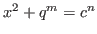with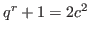, 257-265

### Abstract:

Let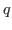be an odd prime, and let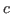,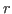be positive integers with. For any nonnegative integer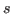, let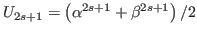and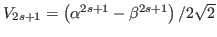, where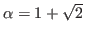and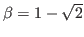. In this paper we prove the following results: (i) If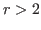, then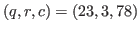and the equation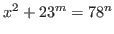has only the positive integer solution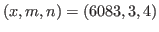. (ii) If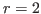and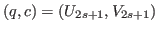with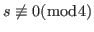, then the equationhas only the positive integer solution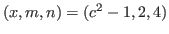.

Key Words: exponential diophantine equation; generalized Ramanujan-Nagell equation; Pell number.

2010 Mathematics Subject Classification: 11D61.